• +91 9971497814
• info@interviewmaterial.com

# RD Chapter 1- Real Numbers Ex-1.6 Interview Questions Answers

### Related Subjects

Question 1 : Without actually performing the long division, state whether the following rational numbers will have a terminating decimal expansion or a non-terminating repeating decimal expansion.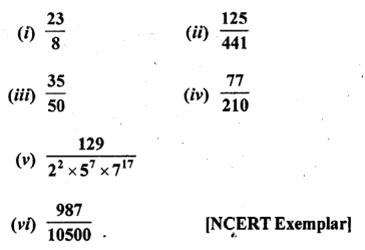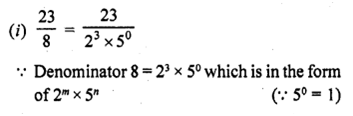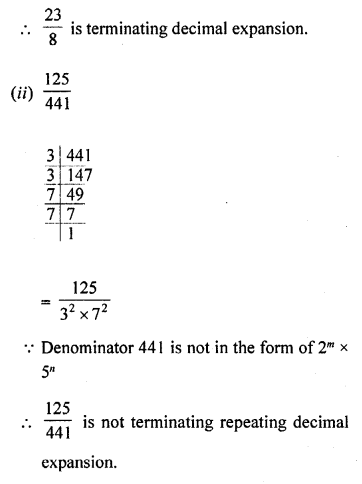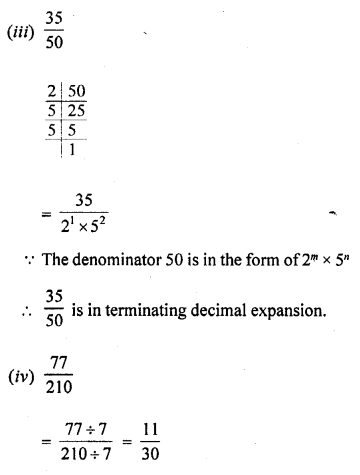Since, the denominator is of the form 2m x5n, the rational number has aterminating decimal expansion.

Question 2 : Write down the decimal expansions of the following rational numbers by writing their denominators in the form 2m x 5n, where m and n are non-negative integers: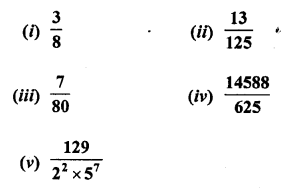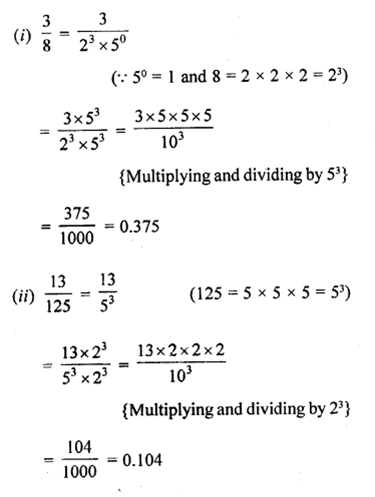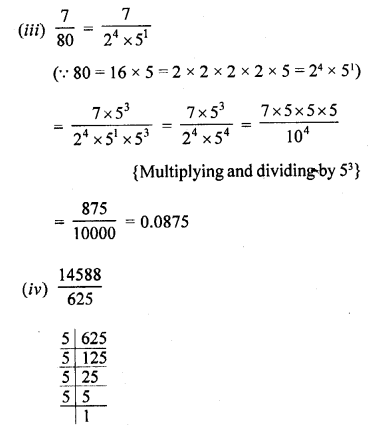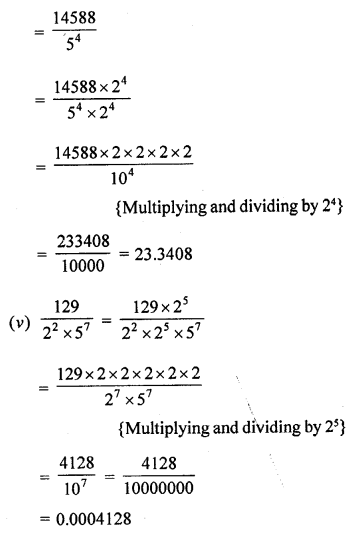Question 3 : Write the denominator of the rational number 257/5000 in the form 2m x 5n , where m, n are non-negative integers. Hence, write the decimal expansion, without actual division.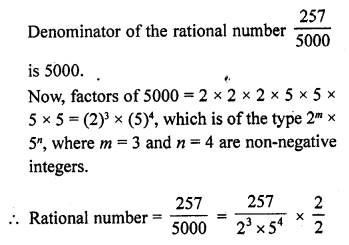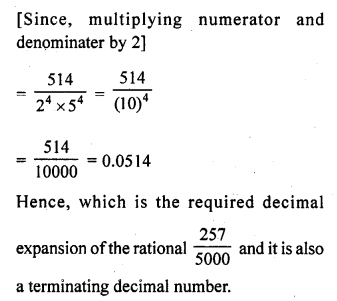Question 4 : What can you say about the prime factorisations of the denominators of the following rationals :

(i) 43.123456789
This decimal fraction is terminating Its denominator will be factorised in theform of 2m x 5n where m and n are non-negativeintegers.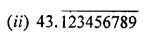This decimal fraction is non-terminating repeating decimals.
The denominator of their fraction will be not in the form of 2m x 5n wherem and n are non-negative integers.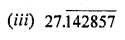This decimal fraction is terminating
Its denominator will be factorised in the form of 2m x5n where m and n are non-negativeintegers
(iv) 0.120120012000120000 ………..
This decimal fraction in non-terminating non recurring
Its denominator will not be factorised in the form of 2m x5n where m and n are non negativeintegers

Question 5 : A rational number in its decimal expansion is 327.7081. What can you say about the prime factors of q, when this number is expressed in the form p/q ? Give reasons. [NCERT Exemplar]

327.7081 is terminating decimal number. So, it represents a rational number and also its denominator must have the form 2m x 5n.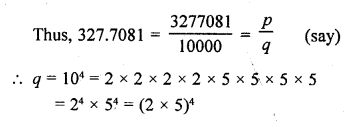Hence, the prime factors of q is 2 and 5.

Todays Deals### RD Chapter 1- Real Numbers Ex-1.6 Contributorskrishan

Name:
Email:

# Latest News# 9000 interview questions in different categories